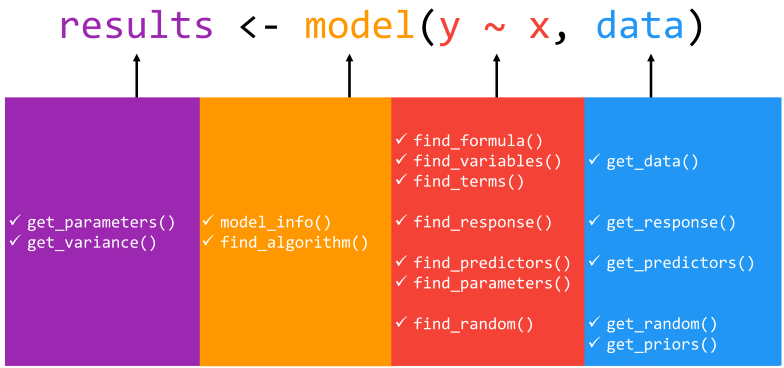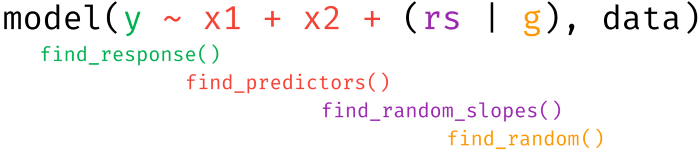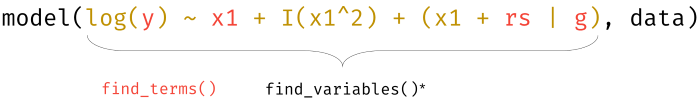Function Design

When fitting any statistical model, there are many useful pieces of information that are simultaneously calculated and stored beyond coefficient estimates and general model fit statistics. Although there exist some generic functions to obtain model information and data, many package-specific modeling functions do not provide such methods to allow users to access such valuable information.

insight is an R-package that fills this important gap by providing a suite of functions to support almost any model. The goal of insight, then, is to provide tools to provide easy, intuitive, and consistent access to information contained in model objects.

Built with non-programmers in mind, insight offers a broad toolbox for making model and data information easily accessible, revolving around two key prefixes: get_* and find_*.

Overview of Core Functions

A statistical model is an object describing the relationship between variables. Although there are a lot of different types of models, each with their specificities, most of them also share some common components. The goal of insight is to help you retrieve these components.

Generally, the get_* prefix extracts values associated with model-specific objects (e.g., parameters or algorithms), while the find_* prefix lists model-specific objects (e.g., priors and predictors). We point users to the package documentation or the complementary package website, https://easystats.github.io/insight/, for a detailed list of the arguments associated with each function as well as the returned values from each function.Definition of Model Components

The functions from insight address different components of a model, however, due to some conceptional overlap, there might be confusion about the specific “targets” of each function. Here is a short explanation how insight defines components of regression models:

• response: the outcome or response variable (dependent variable) of a regression model
• predictor: independent variables of (the fixed part of) a regression model. For mixed models, variables that are (only) in the random effects part of the model are not returned as predictors by default, however, these can be returned using additional arguments to the function call. Predictors are “unqiue”, hence if a variable appears as fixed effect and random slope, it is considered as one predictor (it is the same variable).
• random slopes: variables that are used as random slope in a mixed effects model.
• random or grouping factors: variables that are used as grouping variables in a mixed effects model.
• parameters: values estimated or learned from data that encapsulate the relationship between variables. In regressions, these are usually referred to as coefficients.• term: terms are any (unique) variables that appear in a regression model, like response variable, predictors or random effects. A “term” only relates to the unique occurence of a variable. For instance, in the expression x + I(x^2), there is only the term x.
• variables: A variable is considered as an object that stores unique data information. For instance, the expression x + I(x^2) has two objects with two different sets of data values, and thus are treated as two variables.Examples

Isn’t the predictors, the terms and the parameters the same thing?

In some cases, yes. But not in all cases, and sometimes it is useful to have the “bare” variable names (terms), but sometimes it is also useful to have the information about a possible transformation of variables. That is the main reason for having functions that cover similar aspects of a model object (like find_variables() and find_predictors() or find_terms()).

Here are some examples that demonstrate the differences of each function:

library(insight)
library(lme4)
data(sleepstudy)
sleepstudy$mygrp <- sample(1:5, size = 180, replace = TRUE) sleepstudy$mysubgrp <- NA
sleepstudy$Weeks <- sleepstudy$Days / 7
sleepstudy$cat <- as.factor(sample(letters[1:4], nrow(sleepstudy), replace = TRUE)) for (i in 1:5) { filter_group <- sleepstudy$mygrp == i
sleepstudy$mysubgrp[filter_group] <- sample(1:30, size = sum(filter_group), replace = TRUE) } model <- lmer( Reaction ~ Days + I(Days^2) + log1p(Weeks) + (1 | mygrp / mysubgrp) + (1 + Days | Subject), data = sleepstudy ) # find the response variable find_response(model) #>  "Reaction" # find all predictors, fixed part by default find_predictors(model) #>$conditional
#>  "Days"  "Weeks"

# find random effects, grouping factors only
find_random(model)
#> $random #>  "mysubgrp:mygrp" "mygrp" "Subject" # find random slopes find_random_slopes(model) #>$random
#>  "Days"

# find all predictors, including random effects
find_predictors(model, effects = "all", component = "all")
#> $conditional #>  "Days" "Weeks" #> #>$random
#>  "mysubgrp" "mygrp"    "Subject"

# find all terms, including response and random effects
# this is essentially the same as the previous example plus response
find_terms(model)
#> $response #>  "Reaction" #> #>$conditional
#>  "Days"  "Weeks"
#>
#> $random #>  "mysubgrp" "mygrp" "Subject" # find all variables, i.e. also quadratic or log-transformed predictors find_variables(model) #>$response
#>  "Reaction"
#>
#> $conditional #>  "Days" "I(Days^2)" "log1p(Weeks)" #> #>$random
#>  "mysubgrp" "mygrp"    "mygrp"    "Days"     "Subject"

Finally, there is find_parameters(). Parameters are also known as coefficients, and find_parameters() does exactly that: returning the model coefficients.

# find model parameters, i.e. coefficients
find_parameters(model)
#> $conditional #>  "(Intercept)" "Days" "I(Days^2)" "log1p(Weeks)" #> #>$random
#> $random$mysubgrp:mygrp
#>  "(Intercept)"
#>
#> $random$Subject
#>  "(Intercept)" "Days"
#>
#> $random$mygrp
#>  "(Intercept)"